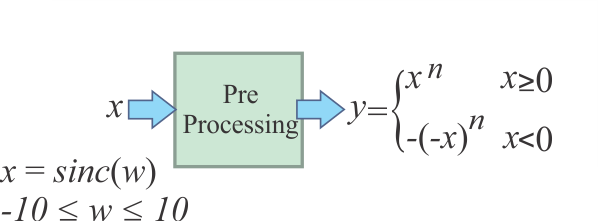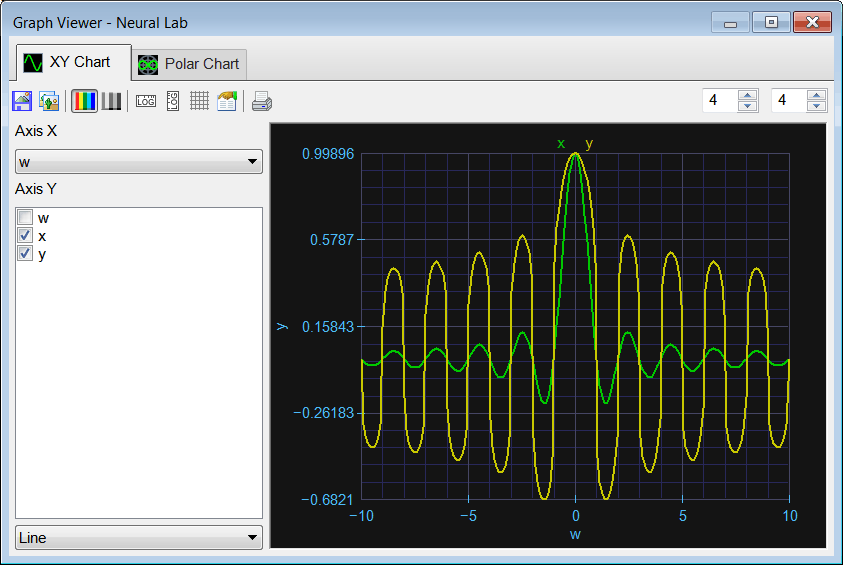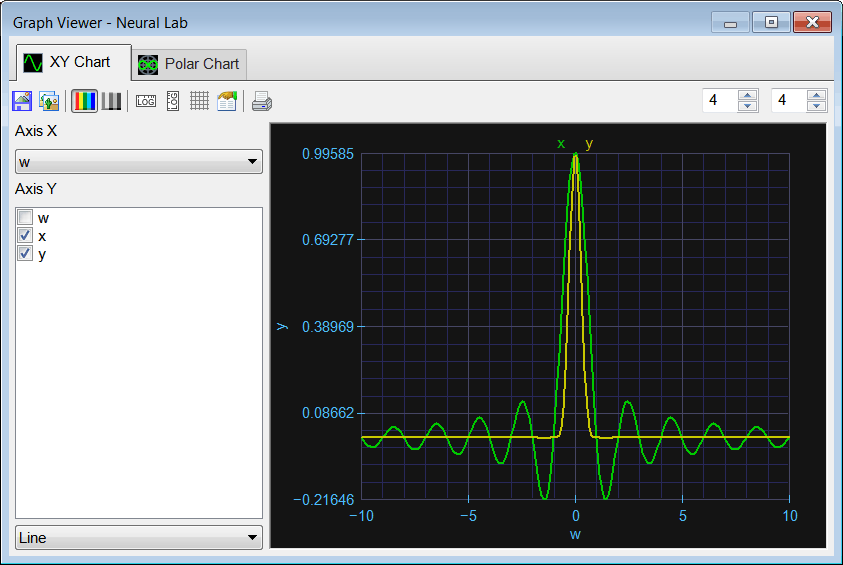# Introduction

## Pre-Processing

In most applications, raw data cannot be applied to an ANN. One easy way to pre-process data is illustrated in the figure. The variable n is called the processing index. The processing index is always positive. From the xy plot, it can be seen that the pre-processing block compress the input signal x for some of its values, and expand x for other values.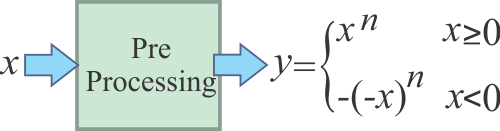Problem 1 Crate a New Project called PrePro to plot the pre-processing function y = f(x) for a processing index n of: 0.25 and 4. (Use the Main file only option.)

 Solution 1 After editing the Main.lab file Run the code by pressing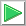Run. You will be asked to save the file, choose an appropriate folder and set the file name to ProcssIndex.lab. Plot the graphs appropriately.

 PrePro\Main.lab Vector x; x.CreateSeries(-1.0, 1.0, 200); Vector y025; Vector y4; y025.Create(200); y4.Create(200); int i = 0; for(i = 0; i<200; i++) {      if (x[i] >= 0.0)      {           y025[i] = pow(x[i], 0.25);           y4[i] = pow(x[i], 4.0);      }      else      {           y025[i] = -pow(-x[i], 0.25);           y4[i] = -pow(-x[i], 4.0);      } }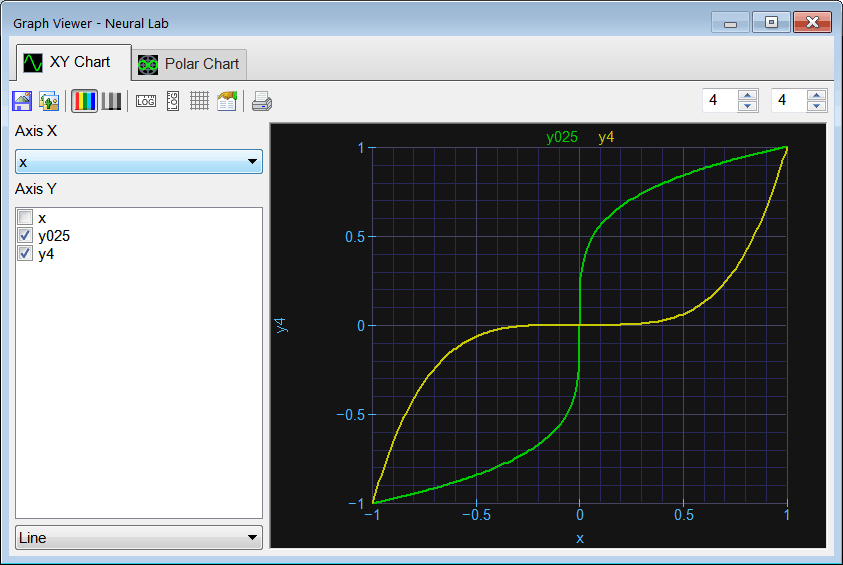Problem 2 (a) When is it appropriate to use a processing index that is less than one? (b) When is it convenient to use a processing index that is bigger than one? (c) What is the purpose of pre-processing the input data of an ANN?

 Problem 3 Consider x = sinc (w) and suppose that x is applied to a pre-processing module (a) Plot y for a processing index of n = 0.25 (ProcssSync.lab file). (b) Plot y for a processing index of n = 4 (ProcssSinc.lab file).